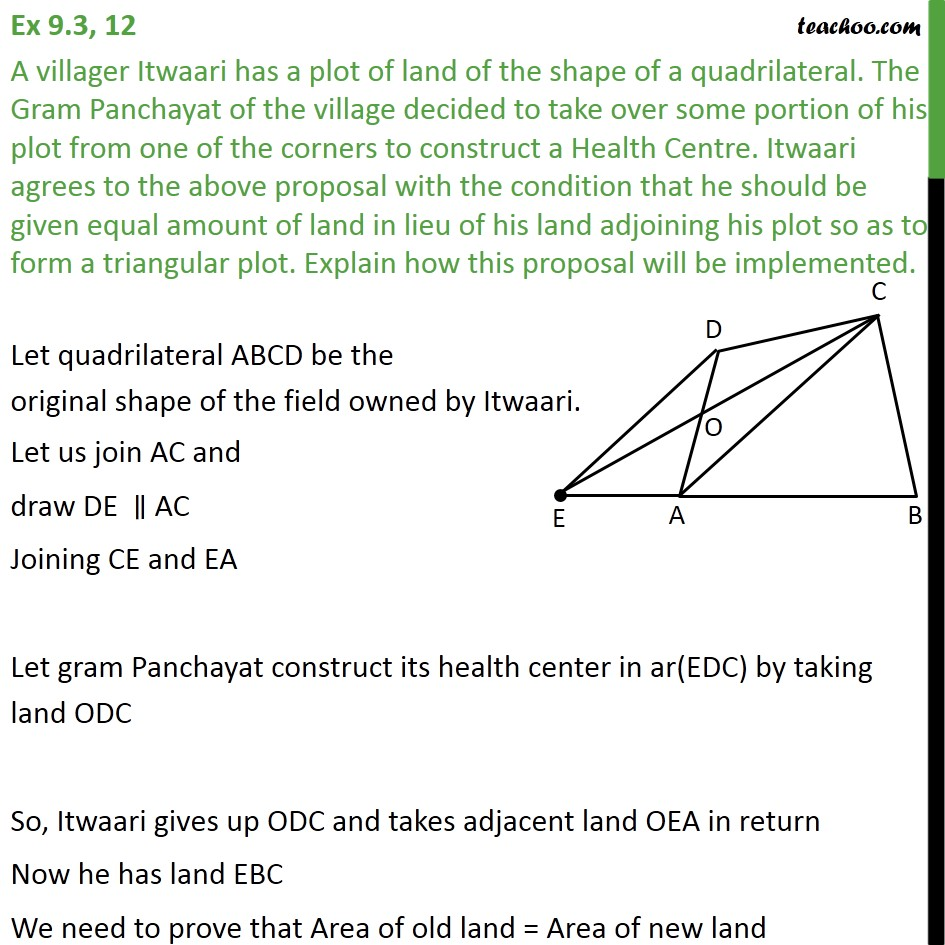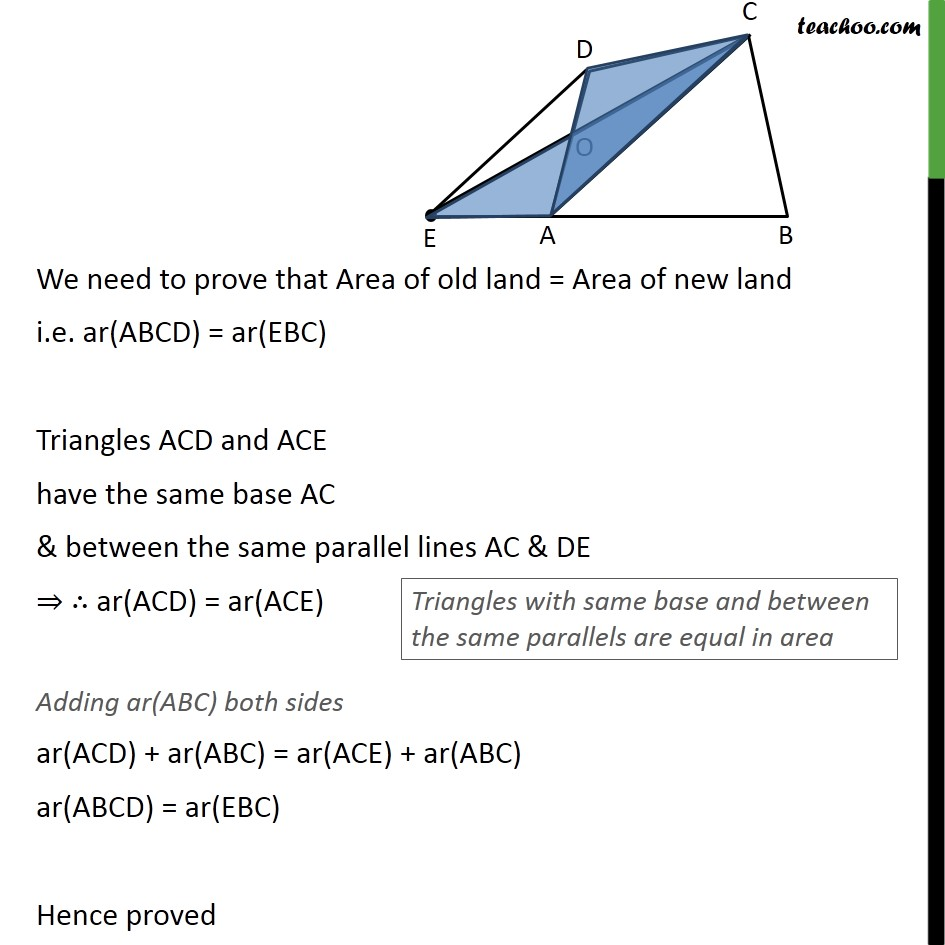Area of Triangles

Areas of Parallelograms and Triangles
Serial order wiseLearn in your speed, with individual attention - Teachoo Maths 1-on-1 Class

### Transcript

Question 12 A villager Itwaari has a plot of land of the shape of a quadrilateral. The Gram Panchayat of the village decided to take over some portion of his plot from one of the corners to construct a Health Centre. Itwaari agrees to the above proposal with the condition that he should be given equal amount of land in lieu of his land adjoining his plot so as to form a triangular plot. Explain how this proposal will be implemented. Let quadrilateral ABCD be the original shape of the field owned by Itwaari. Let us join AC and draw DE ∥ AC Joining CE and EA Let gram Panchayat construct its health center in ar(EDC) by taking land ODC So, Itwaari gives up ODC and takes adjacent land OEA in return Now he has land EBC We need to prove that Area of old land = Area of new land We need to prove that Area of old land = Area of new land i.e. ar(ABCD) = ar(EBC) Triangles ACD and ACE have the same base AC & between the same parallel lines AC & DE ⇒ ∴ ar(ACD) = ar(ACE) Adding ar(ABC) both sides ar(ACD) + ar(ABC) = ar(ACE) + ar(ABC) ar(ABCD) = ar(EBC) Hence proved